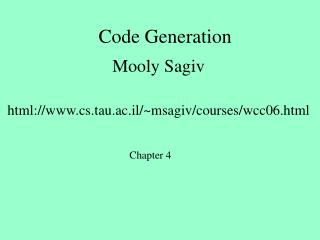DownloadDownload PresentationCode Generation

# Code Generation

Télécharger la présentation## Code Generation

- - - - - - - - - - - - - - - - - - - - - - - - - - - E N D - - - - - - - - - - - - - - - - - - - - - - - - - - -
##### Presentation Transcript

1. Code Generation Mooly Sagiv html://www.cs.tau.ac.il/~msagiv/courses/wcc06.html Chapter 4

2. Tentative Course Schedule

3. Basic Compiler Phases

4. Code Generation • Transform the AST into machine code • Several phases • Many IRs exist • Machine instructions can be described by tree patterns • Replace tree-nodes by machine instruction • Tree rewriting • Replace subtrees • Applicable beyond compilers

5. a := (b[4*c+d]*2)+9

6. movsbl leal

7. Ra + * 9 mem 2 + @b + * Rd 4 Rc

8. Ra + * 9 2 Rt Load_Byte (b+Rd)[Rc], 4, Rt

10. Overall Structure

11. Code generation issues • Code selection • Register allocation • Instruction ordering

12. Simplifications • Consider small parts of AST at time • Simplify target machine • Use simplifying conventions

13. Outline • Simple code generation for expressions (4.2.4, 4.3) • Pure stack machine • Pure register machine • Code generation of basic blocks (4.2.5) • [Automatic generation of code generators (4.2.6)] • Later • Handling control statements • Program Analysis • Register Allocation • Activation frames

14. Simple Code Generation • Fixed translation for each node type • Translates one expression at the time • Local decisions only • Works well for simple machine model • Stack machines (PDP 11, VAX) • Register machines (IBM 360/370) • Can be applied to modern machines

15. Simple Stack Machine SP Stack BP

16. Stack Machine Instructions

17. Example Push_Local #p Push_Const 5 Add_Top2 Store_Local #p p := p + 5

18. Simple Stack Machine Push_Local #p Push_Const 5 Add_Top2 Store_Local #p SP BP+5 7 BP

19. Simple Stack Machine Push_Local #p Push_Const 5 Add_Top2 Store_Local #p SP 7 BP+5 7 BP

20. Simple Stack Machine SP 5 Push_Local #p Push_Const 5 Add_Top2 Store_Local #p 7 BP+5 7 BP

21. Simple Stack Machine Push_Local #p Push_Const 5 Add_Top2 Store_Local #p SP 12 BP+5 7 BP

22. Simple Stack Machine Push_Local #p Push_Const 5 Add_Top2 Store_Local #p SP BP+5 12 BP

23. Register Machine • Fixed set of registers • Load and store from/to memory • Arithmetic operations on register only

24. Register Machine Instructions

25. Example Load_Mem p, R1 Load_Const 5, R2 Add_Reg R2, R1 Store_Reg R1, P p := p + 5

26. Simple Register Machine Load_Mem p, R1 Load_Const 5, R2 Add_Reg R2, R1 Store_Reg R1, P R1 R2 x770 7 memory

27. Simple Register Machine 7 Load_Mem p, R1 Load_Const 5, R2 Add_Reg R2, R1 Store_Reg R1, P R1 R2 x770 7 memory

28. Simple Register Machine 7 5 Load_Mem p, R1 Load_Const 5, R2 Add_Reg R2, R1 Store_Reg R1, P R1 R2 x770 7 memory

29. Simple Register Machine 12 5 Load_Mem p, R1 Load_Const 5, R2 Add_Reg R2, R1 Store_Reg R1, P R1 R2 x770 7 memory

30. Simple Register Machine 12 5 Load_Mem p, R1 Load_Const 5, R2 Add_Reg R2, R1 Store_Reg R1, P R1 R2 x770 12 memory

31. Simple Code Generation for Stack Machine • Tree rewritings • Bottom up AST traversal

32. Abstract Syntax Trees for Stack Machine Instructions

33. Example Subt_Top2 - Mult_Top2 Mult_Top2 * * Mult_Top2 Push_Constant 4 Push_Local #b Push_Local #b b b 4 * a c Push_Local #c Push_Local #a

34. Bottom-Up Code Generation

35. Simple Code Generation forRegister Machine • Need to allocate register for temporary values • AST nodes • The number of machine registers may not suffice • Simple Algorithm: • Bottom up code generation • Allocate registers for subtrees

36. Register Machine Instructions

37. Abstract Syntax Trees forRegister Machine Instructions

38. Simple Code Generation • Assume enough registers • Use DFS to: • Generate code • Assign Registers • Target register • Auxiliary registers

39. Code Generation with Register Allocation

40. Code Generation with Register Allocation(2)

41. Example T=R1 Subt_Reg R1, R2 - T=R2 T=R1 Mult_Reg R3, R2 Mult_Reg R2, R1 * * T=R3 T=R2 Mult_Reg R4, R3 T=R1 T=R2 Load_Constant 4, R2 Load_Mem b, R2 Load_Mem b, R1 b b 4 * T=R4 T=R3 a c Load_Mem c, R4 Load_Mem a, R3

42. Example

43. Runtime Evaluation

44. Optimality • The generated code is suboptimal • May consume more registers than necessary • May require storing temporary results • Leads to larger execution time

45. Example

46. Observation (Aho&Sethi) • The compiler can reorder the computations of sub-expressions • The code of the right-subtree can appear before the code of the left-subtree • May lead to faster code

47. Example T=R1 Subt_Reg R3, R1 - T=R2 T=R1 Mult_Reg R2, R3 Mult_Reg R2, R1 * * T=R2 T=R3 Mult_Reg R3, R2 T=R1 T=R2 Load_Constant 4, R3 Load_Mem b, R2 Load_Mem b, R1 b b 4 * T=R3 T=R2 a c Load_Mem c, R3 Load_Mem a, R2

48. Example Load_Mem b, R1 Load_Mem b, R2 Mult_Reg R2, R1 Load_Mem a, R2 Load_Mem c, R3 Mult_Reg R3, R2 Load_Constant 4, R3 Mult_Reg R2, R3 Subt_Reg R3, R1

49. Two Phase SolutionDynamic ProgrammingSethi & Ullman • Bottom-up (labeling) • Compute for every subtree • The minimal number of registers needed • Weight • Top-Down • Generate the code using labeling by preferring “heavier” subtrees (larger labeling)

50. The Labeling Principle m registers + m > n n registers m registers